# Basic dimensioning

• Dansercoer

#### Dansercoer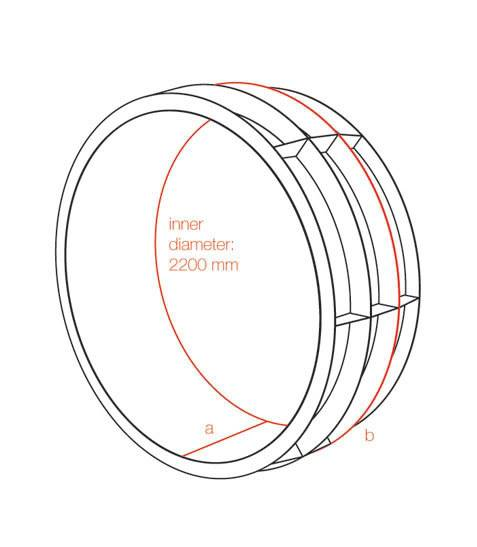Dear all,

Above is a proposal for a human wheel I would like to make, the inner cylinder would be perforated, similar to http://boards.core77.com/viewtopic.php?t=18087 . The difference with a hamster wheel is that it wouldn’t be stationary and is able to take ( large ) bends. The ribs seem rounded, but that’s only an optical illusion. The intention of the wheel is to give an alternative to the walking paths ( paved green space to keep your shoes clean ) in parks, a new experience with more freedom.

I guess the thickness of the materials, the amount of crossbars and the diameter of the outer rings are easiest to decide on the spot. The structure as drawn above would weigh ±80kg in TIG welded aluminium AW-5083 with anti-slip paint, maybe fibreboard is an option too.
But I was wondering how to define the:
- width of the cylinder a
- diameter of the two rings in the middle b

to make sure:
1) the wheel is not too heavy to make it start/stop rolling
2) it is not too difficult to make the wheel lean to one side
3) the wheel is unlikely to fall
( 4)the bends it can take are not too large )

1)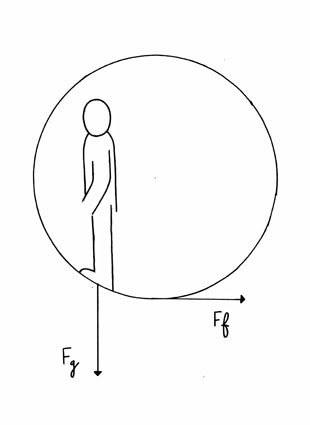The two forces that I think decide whether the wheel is going to roll or not - rolling resistance and gravity on the person - seem to work in different directions?

2)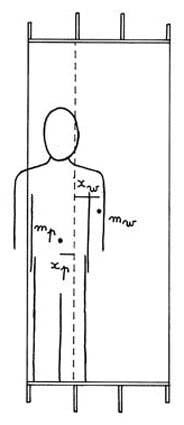The wheel will lean to one side when the combined centre of mass of the wheel and the person in it is not positioned above the resting surface.
(mw.xw - mp.xp) / (mw + mp) = 0
This can be reduced to a function of a and b.

3)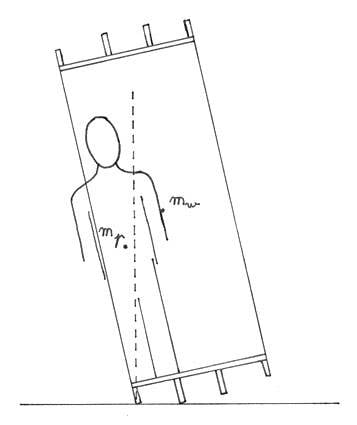The wheel will not fall when the combined centre of mass is positioned above the resting surface.
But what is the influence of inertia - as a result of the leaning movement - on the position of the combined centre of mass?

All of these could result in a graph with a and b as axes from which I could deduct their ideal value.
Parks are not perfectly flat and there might be wind, so I will have to build in safety margins.
The wheel is not intended for hilly parks with high vegetation that limits sight though.

This product designer is thanking you in advance!

If you want it leaning over like that, flare the outer rim out so that it'll be perpendicular to the ground at max cornering. Less chance of it toppling over.

The size of the rings can be found from trigonometry assuming you know the maximum lean angle for cornering. And the width should be as wide as feasably possible to help with stability. I'd make a stab at at least 1.1m wide.

Any why on Earth would you want to make a huge hamster wheel anyway? I'm not bashing, just really curious.

This product designer is thanking you in advance!
For doing his/her job? I bet.

If you want to ask more direct questions and actually do some of the work yourself, you may get better responses.

Thanks for the advice! I could flare the outer rims when the resting surface needs to be a few centimetres wider to achieve balance, I’m just a bit concerned about the price tag and the loss of support for the mesh. I’ll also keep the perforations in the metal mesh smaller than the smallest finger without making it non-transparent.

I’ll have to think about the worst case scenario too; people could deliberately tip over the wheel, there might be a storm (putting the wheel on its side helps) and the angular momentum makes that the braking distance is relatively long...

Last edited:
Dansercoer: On level ground, I think the equation for diagram 1 (your second diagram) would be c > [2*mw*r*a + (mw + mp)*g*b]/(mp*g), where c = horizontal distance from centre of wheel to centroid of human, mw = wheel mass = 80 kg, mp = human mass, r = wheel radius = 1.100 m, a = wheel rectilinear acceleration, b = rolling resistance coefficient (in units of length), and g = 9.8067 m/s^2. I don't have suggestions for example values for b; you will need to research example values. If you want a certain value for a, such as a = 0.1 m/s^2, which means the wheel can start rolling, plug a = 0.1 m/s^2 into the above equation; then the equation says, if c is greater than the right-hand side, the wheel should start rolling (on level ground).

Dansercoer: Your equation for diagram 2 is OK. Parameters a and b are not defined. Alternately, the equation for diagram 2 is, the wheel will lean when xp > mw*xw/mp.

Several more parameters would need to be defined and shown on diagram 3 to develop an equation for that diagram, such as lean angle due to rib height difference, maximum variation in ground surface embedment, human centroid location coordinates, etc. As currently drawn in diagram 3, on a hard ground surface, by inspection, the wheel does not fall over, because both centroids are to the right-hand side of the dashed line.

Last edited:
(mw.xw - mp.xp) / (mw + mp) = 0 is the formula to find the x-component of the combined centre of mass, I took the dotted line as y-axis, so when the x-component is zero we’re at the tipping point.
a and b are not yet defined because I’ll first have to decide on the thickness of the materials, the amount of crossbars and the diameter of the outer rings.

For the third diagram:
The lean angle due to rib height difference (-> b) is what I’m looking for so I can’t define that as a parameter.
As this is a dynamic situation, isn’t there an angular momentum too?

Also, if you have the time, do you mind explaining me how you got to [2*mw*r*a + (mw + mp)*g*b]/(mp*g) ?
I only recognize the rolling resistance force F = [(mw + mp)*g*b]/r in there.

Thanks a lot for the replies!

Dansercoer: For diagram 3, you can ignore angular momentum. For diagram 1, I took summation of moments about the ground contact point, as follows. Summation(M) = 0 = mp*g*c - I*alpha - (mw + mp)*g*b, where I = wheel mass moment of inertia = Io + mw*r^2 = 2*mw*r^2, and alpha = wheel angular acceleration = a/r. Solve for c, then change the equal sign to a greater-than sign. You correctly spotted the rolling resistance force.

Thanks a lot, I think we’re getting there!

I have a few more questions though:
- Isn’t there any angular momentum resulting from the transition from diagram two to three?
- Shouldn’t c be the length of the line between the CM of the person and the ground contact point and shouldn’t gravity be replaced by its component tangent to this line?
- I assume you made the rolling resistance acting on the CM of the wheel? I personally thought this is correct, until somebody made me question it. He told me my rolling resistance should be orientated in the opposite direction acting from the bottom of the wheel in order not to contribute to the rotation of the wheel. I find this very confusing;
http://webphysics.davidson.edu/faculty/dmb/PY430/Friction/rolling.html" [Broken]
-> The first diagram agrees with the above because the wheel is not rolling yet. But down the bottom of the page there’s “distribution of the normal forces creates a net torque negating the rotational contribution of the friction” ?
http://cnx.org/content/m14385/latest/"
-> Here the formulas are completely different (no rolling resistance coefficient) and there’s no deformation.

Last edited by a moderator:
Dansercoer: (1) I didn't attempt to develop an equation for diagram 3. Yes, there is some angular momentum you could include.

(2) Parameter c = horizontal distance from centre of wheel to centroid of human. Human force Fg is essentially downward, same direction as gravity, like you drew it in diagram 1.

(3) I made the rolling resistance a vertically upward force acting at a horizontal distance b in front of the wheel centre ground contact point, which creates a clockwise moment in diagram 1. If you compute a horizontal force couple at the wheel centre and ground necessary to overcome this rolling resistance moment, then the force is F = [(mw + mp)*g*b]/r, pointing forward at the wheel centre, and backward at the ground.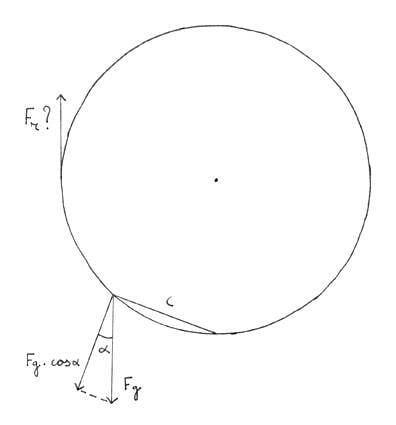This is how I would have calculated the moment created by the person: mp*g*cosα*c
I’m not sure whether I understood the rolling resistance thing very well, you said you “made it act”, can this be done? (assuming you meant r rather than b)
Also, overcoming the rolling resistance isn’t that the task of the person rather than the rolling resistance itself?

Last edited:
I'll change your c to the name c'. Then, mp*g*cos(alpha)*c' = mp*g*c. You can express it either way, because they are equal.

I meant, I drew the rolling resistance force vector vertically upward, at the bottom of the wheel, at a horizontal distance b in front of the wheel centre ground contact point. Rolling resistance coefficient b would generally be much less than r.

Yes, overcoming the rolling resistance is the task of the person. And that's why this overcoming moment is not explicitly included in my moment summation equation. This overcoming moment is already included in m*g*c.

I meant, I drew the rolling resistance force vector vertically upward, at the bottom of the wheel, at a horizontal distance b in front of the wheel centre ground contact point.
I’m sure you’re correct about this, maybe you also know an online source where I could get an understanding of why this is the case?
Also, when I multiply the rolling resistance force with b then I get [(mw + mp)*g*b2]/r

I hope I can find https://www.physicsforums.com/showthread.php?p=2138975" in the first place...

Last edited by a moderator:
The rolling resistance force is (mw + mp)*g. The rolling resistance moment is (mw + mp)*g*b. A horizontal force in a couple, separated by distance r, to overcome the rolling resistance moment would be F = [(mw + mp)*g*b]/r; see the last sentence of post 10. Rolling resistance moment and the moment to overcome the rolling resistance moment are equal and opposite moments. I'm sorry I don't have a good url.